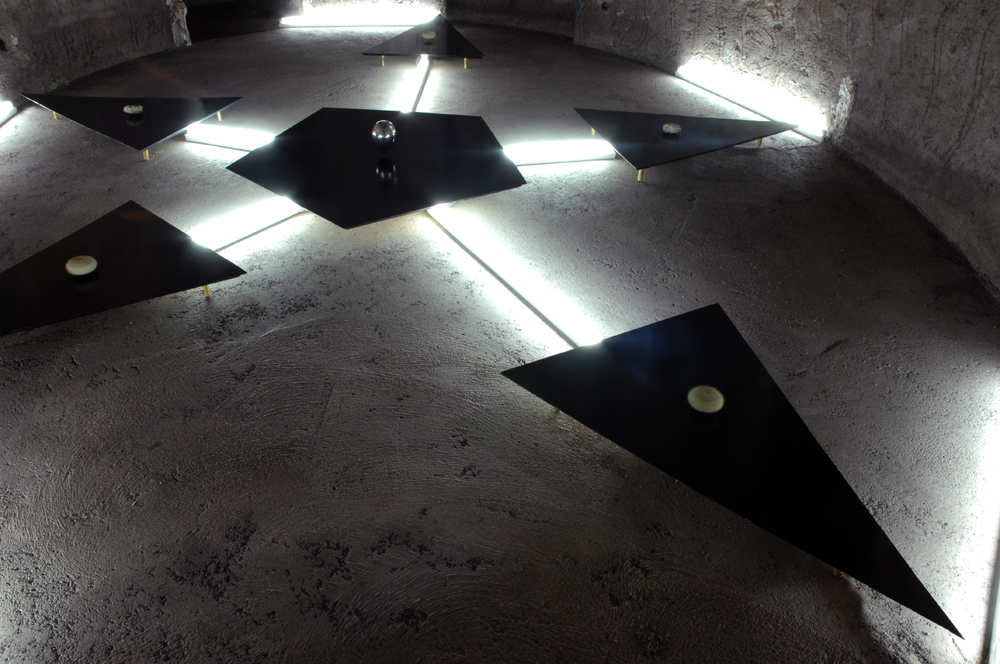THE STAR PLATFORM

February 29 – June 4 – 2012

Marrakech Biennial 4 “Higher Atlas”

Curated by Nadim Samman and Carson Chan.

Theatre Royale, Marrakech.

www.higheratlas.org

The Installation has been realized for the Marrakech Biennial, it is composed by six black platforms connected by a continuous flow of white florescent light, arranged on a total surface of 25 m², in order to keep on fluctuations, five luminescent sponges and one polished aluminum egg.The Star Platform, 2012. Electromagnetic levitation fields, luminescent sponges, aluminium, black Plexiglas, 25 m².

External Reference:

The Barrett–Crane model is a model in quantum gravity which was defined using the Plebanski action.

The$B$ field in the action is supposed to be a$so(3,1)$-valued 2-form, i.e. taking values in the Lie algebra of a special orthogonal group. The term$B^{ij}\wedge B^{kl}$

in the action has the same symmetries as it does to provide the Einstein–Hilbert action. But the form of$B^{ij}$

is not unique and can be posed by the different forms:

•$\pm e^{i}\wedge e^{j}$
•$\pm \epsilon ^{ijkl}e_{k}\wedge e_{l}$

where$e^{i}$ is the tetrad and$\epsilon ^{ijkl}$ is the antisymmetric symbol of the$so(3,1)$-valued 2-form fields.

The Plebanski action can be constrained to produce the BF model which is a theory of no local degrees of freedomJohn W. Barrett and Louis Crane modeled the analogous constraint on the summation over spin foam.

The Barrett–Crane model on spin foam quantizes the Plebanski action, but its path integral amplitude corresponds to the degenerate$B$ field and not the specific definition$B^{ij}=e^{i}\wedge e^{j}$,

which formally satisfies the Einstein’s field equation of general relativity. However, if analysed with the tools of loop quantum gravity the Barrett–Crane model gives an incorrect long-distance limit , and so the model is not identical to loop quantum gravity.